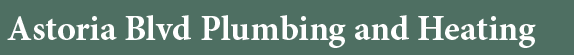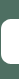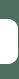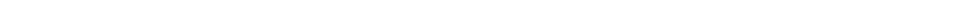## Heating

Sizing an Electric Room Heater:

Once you decide what type of electric heater you want to use, the next step is determining what size unit you need.
Electric heaters come in a range of sizes measured in how much energy they consume and heat they put out, and are rated in Watts.
To size an electrical heater for a room, first understand that the size will be affected by heat loss through the walls and windows in the room. So the amount of insulation and quality and number of windows will have an effect on the heater size.

However, as a rough rule of thumb, assume the heater to be sized at 10 watts per square foot of room being heated. As the graphic above shows, measure the length of the room and the width of the room. Multiply the lengths times the width and then multiply that by 10. This will give you the rough wattage for the heater. If the room is poorly insulated or has a lot of windows, select a slightly larger unit.

 Our Services